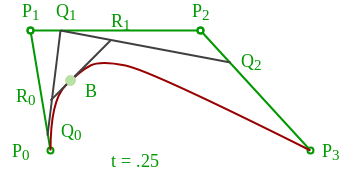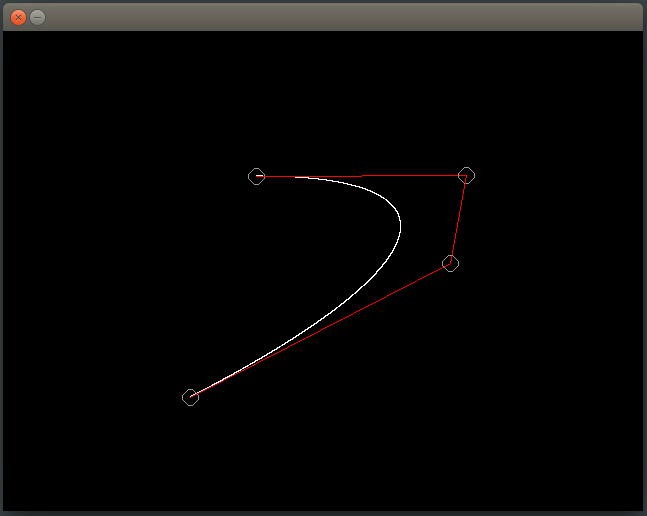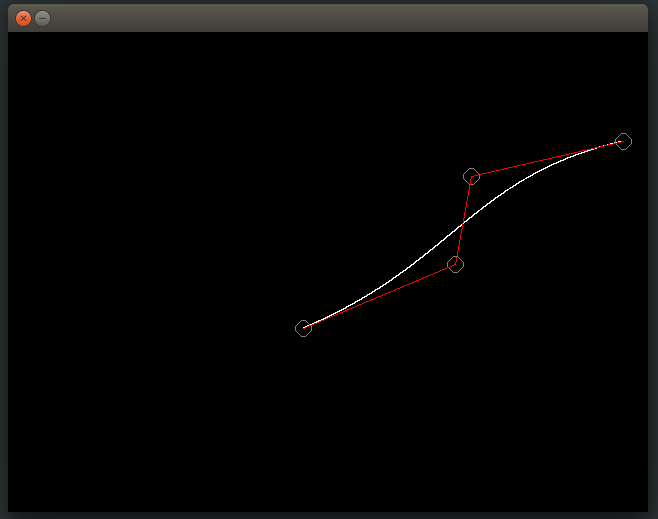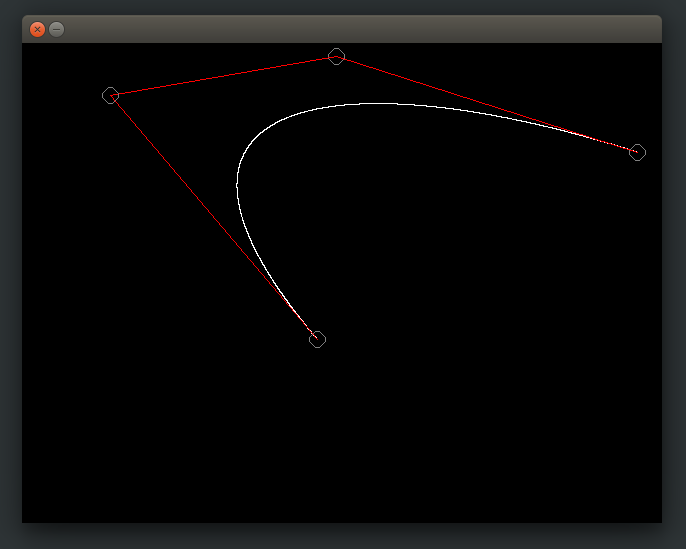# Cubic Bezier Curve Implementation in C

What is a bezier curve?
So a Bezier curve is a mathematically defined curve used in two-dimensional graphic applications like abode illustrator,inkscape etc.The curve is defined by four points: the initial position and the terminating position i.e P0 and P3 respectively (which are called “anchors”) and two separate middle points i.e P1 and P2(which are called “handles”) in our example.Bezier curves are frequently used in computer graphics, animation, modeling etc.

How do we Represent Bezier Curves Mathematically ?
Bezier curves can be generated under the control of other points. Approximate tangents by using control points are used to generate curve. The Bezier curve can be represented mathematically as –Whereis the set of points andrepresents the Bernstein polynomials i.e. Blending Function which are given by –Where n is the polynomial order, i is the index, and u/t is the variable which have from 0 to 1.

Let us define our cubic bezier curve mathematically.
So a bezier curve id defined by a set of control pointstowhere n is called its order(n = 1 for linear , n = 2 for quadratic , etc.). The first and last control points are always the end points of the curve; however, the intermediate control points (if any) generally do not lie on the curve.
For cubic bezier curve order(n) of polynomial is 3 , index(i) vary from i = 0 to i = n i.e. 3 and u will vary from.

Cubic Bezier Curve function is defined as :Cubic Bezier Curve blending function are defined as :SoandNow,So we will calculate curve x and y pixel by incrementing value of u by 0.0001.Construction of a cubic Bézier curve

Properties of bezier curves

1. They always pass through the first and last control points.

2. They are contained in the convex hull of their defining control points.

3. The degree of the polynomial defining the curve segment is one less that the number of defining polygon point. Therefore, for 4 control points, the degree of the polynomial is 3, i.e. cubic polynomial.

4. A Bezier curve generally follows the shape of the defining polygon

5. The direction of the tangent vector at the end points is same as that of the vector determined by first and last segments.

6. Bezier curves exhibit global control means moving a control point alters the shape of the whole curve

NOTE:The following implementation uses SDL library to draw pixels on screen . If you are on debian system like ubuntu just run following command to install SDL library.

sudo apt-get install libsdl2-dev

To build use

gcc fileName.c -lSDL2 -lm

 // C program to implement   // Cubic Bezier Curve      /* install SDL library for running thing code*/ /* install by using this commamnd line : sudo apt-get install libsdl2-dev */ /* run this code using command : gcc fileName.c -lSDL2 -lm*/    #include  #include  #include  #include     SDL_Window* window = NULL;  SDL_Renderer* renderer = NULL;  int mousePosX , mousePosY ;  int xnew , ynew ;     /*Function to draw all other 7 pixels present at symmetric position*/ void drawCircle(int xc, int yc, int x, int y)  {      SDL_RenderDrawPoint(renderer,xc+x,yc+y) ;      SDL_RenderDrawPoint(renderer,xc-x,yc+y);      SDL_RenderDrawPoint(renderer,xc+x,yc-y);      SDL_RenderDrawPoint(renderer,xc-x,yc-y);      SDL_RenderDrawPoint(renderer,xc+y,yc+x);      SDL_RenderDrawPoint(renderer,xc-y,yc+x);      SDL_RenderDrawPoint(renderer,xc+y,yc-x);      SDL_RenderDrawPoint(renderer,xc-y,yc-x);  }     /*Function for circle-generation using Bresenham's algorithm */ void circleBres(int xc, int yc, int r)  {      int x = 0, y = r;      int d = 3 - 2 * r;      while (y >= x)      {          /*for each pixel we will draw all eight pixels */         drawCircle(xc, yc, x, y);          x++;             /*check for decision parameter and correspondingly update d, x, y*/         if (d > 0)          {              y--;              d = d + 4 * (x - y) + 10;          }          else             d = d + 4 * x + 6;          drawCircle(xc, yc, x, y);      }  }     /* Function that take input as Control Point x_coordinates and   Control Point y_coordinates and draw bezier curve */ void bezierCurve(int x[] , int y[])  {      double xu = 0.0 , yu = 0.0 , u = 0.0 ;      int i = 0 ;      for(u = 0.0 ; u <= 1.0 ; u += 0.0001)      {          xu = pow(1-u,3)*x+3*u*pow(1-u,2)*x+3*pow(u,2)*(1-u)*x               +pow(u,3)*x;          yu = pow(1-u,3)*y+3*u*pow(1-u,2)*y+3*pow(u,2)*(1-u)*y              +pow(u,3)*y;          SDL_RenderDrawPoint(renderer , (int)xu , (int)yu) ;      }  }  int main(int argc, char* argv[])  {      /*initialize sdl*/     if (SDL_Init(SDL_INIT_EVERYTHING) == 0)      {          /*              This function is used to create a window and default renderer.              int SDL_CreateWindowAndRenderer(int width                                             ,int height                                            ,Uint32 window_flags                                             ,SDL_Window** window                                             ,SDL_Renderer** renderer)              return 0 on success and -1 on error          */         if(SDL_CreateWindowAndRenderer(640, 480, 0, &window, &renderer) == 0)          {              SDL_bool done = SDL_FALSE;                 int i = 0 ;              int x , y , flagDrawn = 0 ;                 while (!done)              {                  SDL_Event event;                     /*set background color to black*/                 SDL_SetRenderDrawColor(renderer, 0, 0, 0, SDL_ALPHA_OPAQUE);                  SDL_RenderClear(renderer);                     /*set draw color to white*/                 SDL_SetRenderDrawColor(renderer, 255, 255, 255, SDL_ALPHA_OPAQUE);                     /* We are drawing cubic bezier curve                   which has four control points */                 if(i==4)                  {                      bezierCurve(x , y) ;                      flagDrawn = 1 ;                  }                     /*grey color circle to encircle control Point P0*/                 SDL_SetRenderDrawColor(renderer, 128, 128, 128, SDL_ALPHA_OPAQUE);                  circleBres(x , y , 8) ;                     /*Red Line between control Point P0 & P1*/                 SDL_SetRenderDrawColor(renderer, 255, 0, 0, SDL_ALPHA_OPAQUE);                  SDL_RenderDrawLine(renderer , x , y , x , y) ;                     /*grey color circle to encircle control Point P1*/                 SDL_SetRenderDrawColor(renderer, 128, 128, 128, SDL_ALPHA_OPAQUE);                  circleBres(x , y , 8) ;                     /*Red Line between control Point P1 & P2*/                 SDL_SetRenderDrawColor(renderer, 255, 0, 0, SDL_ALPHA_OPAQUE);                  SDL_RenderDrawLine(renderer , x , y , x , y) ;                     /*grey color circle to encircle control Point P2*/                 SDL_SetRenderDrawColor(renderer, 128, 128, 128, SDL_ALPHA_OPAQUE);                  circleBres(x , y , 8) ;                     /*Red Line between control Point P2 & P3*/                 SDL_SetRenderDrawColor(renderer, 255, 0, 0, SDL_ALPHA_OPAQUE);                  SDL_RenderDrawLine(renderer , x , y , x , y) ;                     /*grey color circle to encircle control Point P3*/                 SDL_SetRenderDrawColor(renderer, 128, 128, 128, SDL_ALPHA_OPAQUE);                  circleBres(x , y , 8) ;                     /*We are Polling SDL events*/                 if (SDL_PollEvent(&event))                  {                      /* if window cross button clicked then quit from window */                     if (event.type == SDL_QUIT)                      {                          done = SDL_TRUE;                      }                      /*Mouse Button is Down */                     if(event.type == SDL_MOUSEBUTTONDOWN)                      {                          /*If left mouse button down then store                             that point as control point*/                         if(event.button.button == SDL_BUTTON_LEFT)                          {                              /*store only four points                               because of cubic bezier curve*/                             if(i < 4)                              {                                  printf("Control Point(P%d):(%d,%d)\n"                                  ,i,mousePosX,mousePosY) ;                                     /*Storing Mouse x and y positions                                   in our x and y coordinate array */                                 x[i] = mousePosX ;                                  y[i] = mousePosY ;                                  i++ ;                              }                          }                      }                      /*Mouse is in motion*/                     if(event.type == SDL_MOUSEMOTION)                      {                          /*get x and y positions from motion of mouse*/                         xnew = event.motion.x ;                          ynew = event.motion.y ;                             int j ;                             /* change coordinates of control point                            after bezier curve has been drawn */                         if(flagDrawn == 1)                          {                              for(j = 0 ; j < i ; j++)                              {                                  /*Check mouse position if in b/w circle then                             change position of that control point to mouse new                                   position which are coming from mouse motion*/                                 if((float)sqrt(abs(xnew-x[j]) * abs(xnew-x[j])                                        + abs(ynew-y[j]) * abs(ynew-y[j])) < 8.0)                                  {                                      /*change coordinate of jth control point*/                                     x[j] = xnew ;                                      y[j] = ynew ;                                      printf("Changed Control Point(P%d):(%d,%d)\n"                                            ,j,xnew,ynew) ;                                  }                              }                          }                          /*updating mouse positions to positions                           coming from motion*/                         mousePosX = xnew ;                          mousePosY = ynew ;                      }                  }                  /*show the window*/                 SDL_RenderPresent(renderer);              }          }          /*Destroy the renderer and window*/         if (renderer)          {              SDL_DestroyRenderer(renderer);          }          if (window)          {              SDL_DestroyWindow(window);          }      }      /*clean up SDL*/     SDL_Quit();      return 0;  }

Output

Move mouse when mouse position is b/w circle then only curve shape will be changedAttention reader! Don’t stop learning now. Get hold of all the important DSA concepts with the DSA Self Paced Course at a student-friendly price and become industry ready.

My Personal Notes arrow_drop_upIf you like GeeksforGeeks and would like to contribute, you can also write an article using contribute.geeksforgeeks.org or mail your article to contribute@geeksforgeeks.org. See your article appearing on the GeeksforGeeks main page and help other Geeks.

Please Improve this article if you find anything incorrect by clicking on the "Improve Article" button below.

Improved By : ManasChhabra2

Article Tags :
Practice Tags :

3

Please write to us at contribute@geeksforgeeks.org to report any issue with the above content.